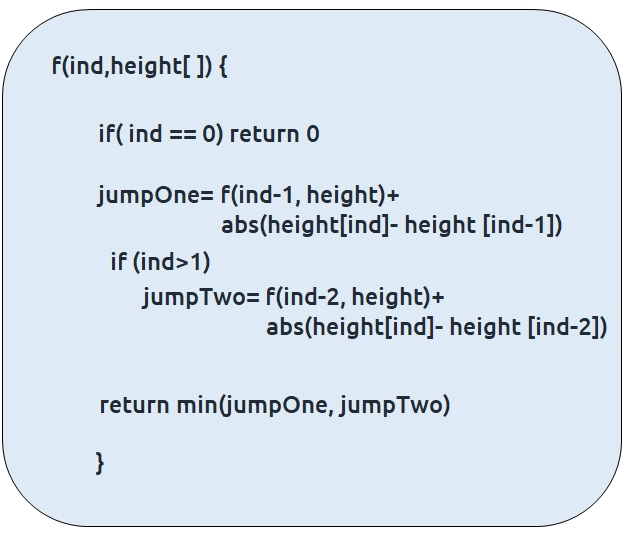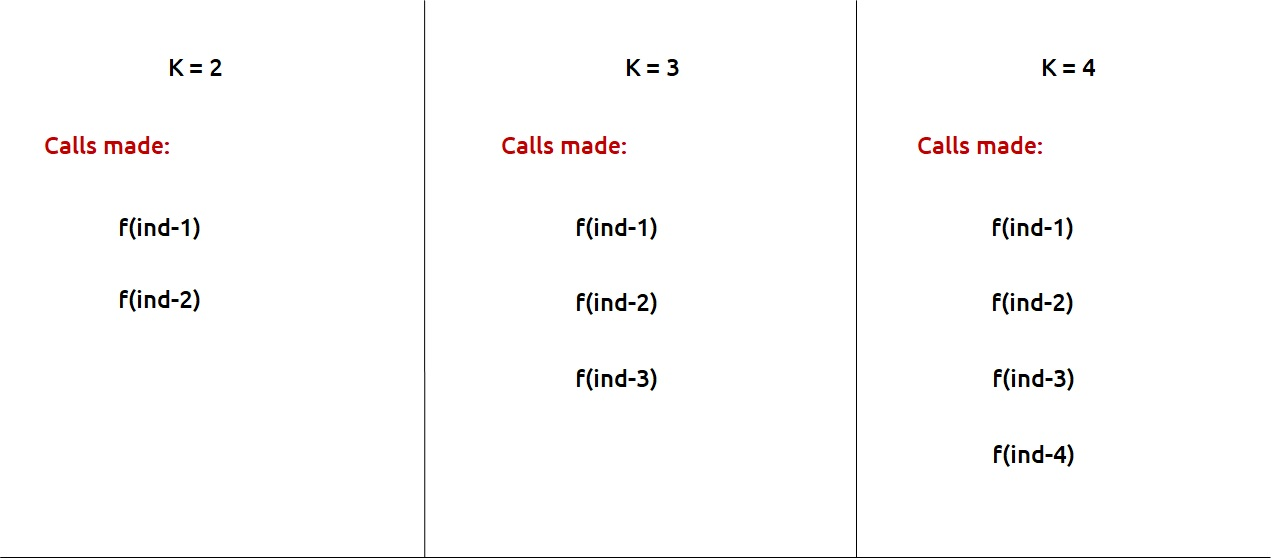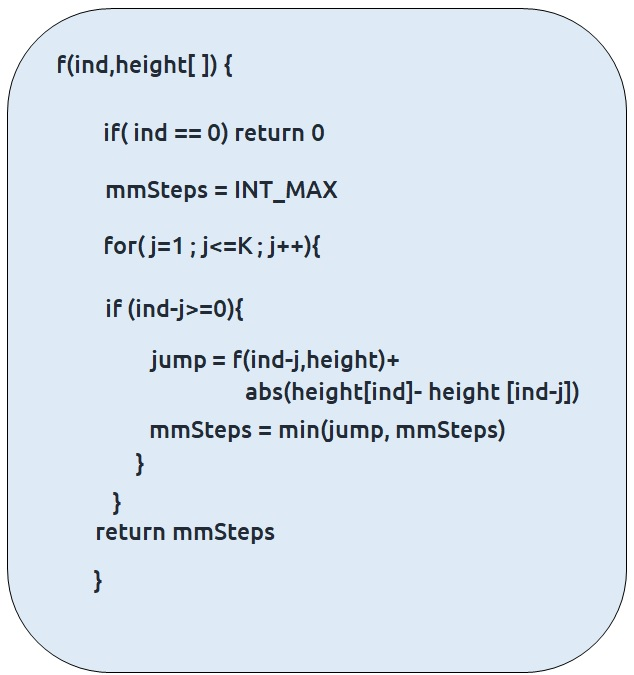# Dynamic Programming: Frog Jump with k Distances (DP 4)

In this article we will learn about “Dynamic Programming: Frog Jump with k Distances (DP 4)”

Problem Statement:  Frog Jump with K Distance/ Learn to write 1D DP

Problem Statement:

This is a follow-up question to “Frog Jump” discussed in the previous article. In the previous question, the frog was allowed to jump either one or two steps at a time. In this question, the frog is allowed to jump up to ‘K’ steps at a time. If K=4, the frog can jump 1,2,3, or 4 steps at every index.

Pre-req: Frog Jump

### Solution :

We will first see the modifications required in the pseudo-code. Once the recursive code is formed, we can go ahead with the memoization and tabulation.

Here is the pseudocode from the simple Frog Jump problem.This was the case where we needed to try two options (move a single step and move two steps) in order to try out all the possible ways for the problem. Now, we need to try K options in order to try out all possible ways.

These are the calls we need to make for K=2, K=3, K=4If we generalize, we are making K calls, therefore, we can set a for loop to run from 1 to K and in each iteration we can make a function call, corresponding to a step. We will return the minimum step call after the loop.

The final pseudo-code will be:Note: We need to make sure that we are not passing negative index to the array, therefore an extra if the condition is used.

Once we form the recursive solution, we can use the approach told in Dynamic Programming Introduction to convert it into a dynamic programming one.

Memoization approach

Steps to convert Recursive code to memoization solution:

• Create a dp[n] array initialized to -1.
• Whenever we want to find the answer of a particular value (say n), we first check whether the answer is already calculated using the dp array(i.e dp[n] != -1 ). If yes, simply return the value from the dp array.
• If not, then we are finding the answer for the given value for the first time, we will use the recursive relation as usual but before returning from the function, we will set dp[n] to the solution we get.

Code:

## C++ Code

``````#include <bits/stdc++.h>

using namespace std;

int solveUtil(int ind, vector<int>& height, vector<int>& dp, int k){
if(ind==0) return 0;
if(dp[ind]!=-1) return dp[ind];

int mmSteps = INT_MAX;

for(int j=1;j<=k;j++){
if(ind-j>=0){
int jump = solveUtil(ind-j, height, dp, k)+ abs(height[ind]- height[ind-j]);
mmSteps= min(jump, mmSteps);
}
}
return dp[ind]= mmSteps;

}

int solve(int n, vector<int>& height , int k){
vector<int> dp(n,-1);
return solveUtil(n-1, height, dp, k);
}

int main() {

vector<int> height{30,10,60 , 10 , 60 , 50};
int n=height.size();
int k=2;
vector<int> dp(n,-1);
cout<<solve(n,height,k);
}

``````

Output: 40

Time Complexity: O(N *K)

Reason: The overlapping subproblems will return the answer in constant time. Therefore the total number of new subproblems we solve is ‘n’. At every new subproblem, we are running another loop for K times. Hence total time complexity is O(N * K).

Space Complexity: O(N)

Reason: We are using a recursion stack space(O(N)) and an array (again O(N)). Therefore total space complexity will be O(N) + O(N) ≈ O(N)

## Java Code

``````import java.util.*;
class TUF{
static int solveUtil(int ind, int[] height, int[] dp, int k){
if(ind==0) return 0;
if(dp[ind]!=-1) return dp[ind];

int mmSteps = Integer.MAX_VALUE;

for(int j=1;j<=k;j++){
if(ind-j>=0){
int jump = solveUtil(ind-j, height, dp, k)+ Math.abs(height[ind]-
height[ind-j]);
mmSteps= Math.min(jump, mmSteps);
}
}
return dp[ind]= mmSteps;

}

static int solve(int n, int[] height , int k){
int dp[]=new int[n];
Arrays.fill(dp,-1);
return solveUtil(n-1, height, dp, k);
}

public static void main(String args[]) {

int height[]={30,10,60 , 10 , 60 , 50};
int n=height.length;
int k=2;
System.out.println(solve(n,height,k));
}
}

``````

Output: 40

Time Complexity: O(N *K)

Reason: The overlapping subproblems will return the answer in constant time. Therefore the total number of new subproblems we solve is ‘n’. At every new subproblem, we are running another loop for K times. Hence total time complexity is O(N * K).

Space Complexity: O(N)

Reason: We are using a recursion stack space(O(N)) and an array (again O(N)). Therefore total space complexity will be O(N) + O(N) ≈ O(N)

Tabulation approach

• Declare a dp[] array of size n.
• First initialize the base condition values, i.e dp as 0.
• Set an iterative loop which traverses the array( from index 1 to n-1) and for every index calculate jumpOne and jumpTwo and set dp[i] = min(jumpOne, jumpTwo).

Code:

## C++ Code

``````#include <bits/stdc++.h>

using namespace std;

int solveUtil(int n, vector<int>& height, vector<int>& dp, int k){
dp=0;
for(int i=1;i<n;i++){
int mmSteps = INT_MAX;

for(int j=1;j<=k;j++){
if(i-j>=0){
int jump = dp[i-j]+ abs(height[i]- height[i-j]);
mmSteps= min(jump, mmSteps);
}
}
dp[i]= mmSteps;
}
return dp[n-1];
}

int solve(int n, vector<int>& height , int k){
vector<int> dp(n,-1);
return solveUtil(n, height, dp, k);
}

int main() {

vector<int> height{30,10,60 , 10 , 60 , 50};
int n=height.size();
int k=2;
vector<int> dp(n,-1);
cout<<solve(n,height,k);
}
``````

Output: 40

Time Complexity: O(N*K)

Reason: We are running two nested loops, where outer loops run from 1 to n-1 and the inner loop runs from 1 to K

Space Complexity: O(N)

Reason: We are using an external array of size ‘n’’.

## Java Code

``````import java.util.*;
class TUF{
static int solveUtil(int n, int[] height, int[] dp, int k){
dp=0;
for(int i=1;i<n;i++){
int mmSteps = Integer.MAX_VALUE;

for(int j=1;j<=k;j++){
if(i-j>=0){
int jump = dp[i-j]+ Math.abs(height[i]- height[i-j]);
mmSteps= Math.min(jump, mmSteps);
}
}
dp[i]= mmSteps;
}
return dp[n-1];

}

static int solve(int n, int[] height , int k){
int dp[]=new int[n];
Arrays.fill(dp,-1);
return solveUtil(n, height, dp, k);
}

public static void main(String args[]) {

int height[]={30,10,60 , 10 , 60 , 50};
int n=height.length;
int k=2;
System.out.println(solve(n,height,k));
}
}

``````

Output: 40

Time Complexity: O(N*K)

Reason: We are running two nested loops, where outer loops run from 1 to n-1 and the inner loop runs from 1 to K

Space Complexity: O(N)

Reason: We are using an external array of size ‘n’’.

Special thanks to Anshuman Sharma for contributing to this article on takeUforward. If you also wish to share your knowledge with the takeUforward fam, please check out this article